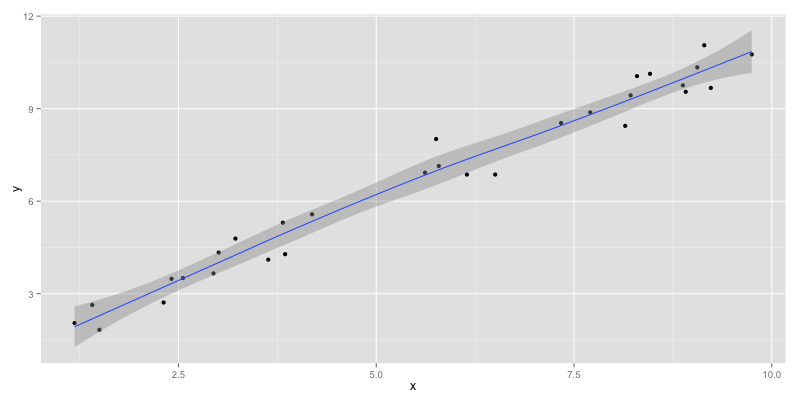# Deriving the Predicted Residual Sum of Squares Statistic

Recently I was looking into measures to evaluate a regularized least squares model. One thing I would have liked was cross-validation to be able to compare different models. When researching possibilities, I discovered PRESS (Predicted Residual Sum of Squares Statistic).

The main resources I used to study the subject are:

There are also some online resources (they don’t provide mathematical background but R code):

The PRESS is similar to the Leave-One-Out-Cross-Validation (LOOCV) where each sample is in turn used as a test sample. For linear least squares there is a simple well known non-iterative formula to compute the PRESS. This should be extended to fit more complex least squares objectives (e.g. with a regularization matrix). In this post I would like to show you how to derive a closed form computation (approximation!) for the PRESS statistic as shown in  and .

### The objective

The model with targets (y), observations (X) and model (\beta) is

$$g(X; \beta) = X \beta$$

Therefore the objective of our regularized least squares with regularization matrix (\Pi) is

$$\min_{\beta} \left[\beta^T \Pi \beta + (y – X \beta)^T (y – X \beta)\right]$$

This regularization term is simplified from what was described in . Bartoli also uses a smoothing parameter (\mu) which influences (\Pi) and has to be optimized as well, which is leading to a nested optimization problem. In our case we use a diagonal matrix with small entries (e.g. 0.01) as regularization term.

The solution for this minimization problem is

$$\beta^* = \left[X \Pi + X^T X\right]^{-1} X^T y$$

### Iterative LOOCV

The Leave-One-Out-Cross-Validation score for this problem can be defined as:

$$\epsilon^2 = \frac{1}{N} \sum\limits_{j=1}^N || g(X_j; \beta^j) – y_j ||$$

where

• N is the number of elements (samples) in X
• $$\beta^j$$ is the model as if trained without the j-th sample
• $$g(X_j; \beta^j)$$ is the prediction on the j-th sample which is an unseen sample (since the model is trained without it)

Note that this score is computed in an iterative manner which might be very time consuming since you have to train N different models.

### Deriving the PRESS

In a first step we define (y^j) which is (y) without the j-th entry. Therefore we use (e_j) (a vector of zeroes and a one at the j-th position).

$$y^j = y – e_j (y_j – \beta^T X)$$

This is like replacing the j-th target value by the prediction.

We also define a matrix T (compare it with (\beta^*) – the only difference is y):

$$T(N) = \left[N \Pi + X^T X\right]^{-1} X^T$$

This influence matrix T maps the targets (y) to the model coefficients (\beta^*).

As shown in , the model coefficients when removing the j-th entry can be written as:

$$\beta^{j} = T(N-1) y^j$$

We define another influence matrix that maps the targets to the predicted ones:

$$H(\gamma) = XT(\gamma) = X(X^TX + \gamma \Pi^T \Pi) ^ {-1} X^T$$

H is a symmetric (N \times N) matrix, which means it has as many rows and columns as there are targets. To refer to the j-th column (or row) we can write (h_j). Therefore we can write:

$$g(X_j; \beta) = y^T h_j(N)$$

$$g(X_j; \beta^j) = (y^j)^T h_j(N-1)$$

We take the difference between those two equations:

$$g(X_j; \beta)-g(X_j; \beta^j) = y^T h_j(N)-(y^j)^T h_j(N-1)$$

By approximating (h_j = h_j(N) \approx h_j(N-1)) we can write:

$$g(X_j; \beta)-g(X_j; \beta^j) \approx (y – y^j)^T h_j$$

Substituting (y^j) with its definition from above leads to:

$$g(X_j; \beta)-g(X_j; \beta^j) \approx h_j^T e_j (y_j – g(X_j; \beta^j))$$

Using (h_{j,j} = h_j^Te_j), the diagonal elements of (H(N)) we get:

$$g(X_j; \beta)-g(X_j; \beta^j) \approx h_{j,j} (y_j – g(X_j; \beta^j))$$

We can rearrange this to:

$$h_{j,j}y_j + (1 – h_{j,j}) g(X_j; \beta^j) \approx g(X_j; \beta)$$

Then we subtract (y_j) on both sides.

$$h_{j,j} y_j + (1 – h_{j,j}) g(X_j; \beta^j) – y_j \approx g(X_j; \beta) – y_j$$

$$(1 – h_{j,j}) (g(X_j; \beta^j) – y_j) \approx g(X_j; \beta^j) – y_j$$

This leads to an analytical, non-iterative expression rewritten in matrix form:

$$\epsilon^2 \approx \frac{1}{m} \left|\left|diag\left(\frac{1}{\mathbf{1}-diag(H(m))}\right)(X\beta-y)\right|\right|^2$$

That’s it, we have derived a closed form for the PRESS 🙂

### Important notes

Some points that should be considered:

• The computation of the PRESS is an approximation
• The smaller the PRESS, the better
• The approximations $$h_j = h_j(N) \approx h_j(N-1)$$ are called N- respectively N-1-approximation. It is necessary to be able to derive a closed form. Bartoli conclude that there is no significant difference between the two approximations.